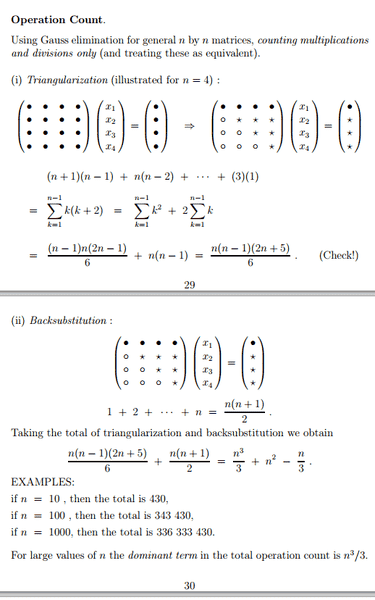# Operation Count w/ LU Decomposition

• twoski
In summary, the total multiplications and divisions needed for the LU Decomp. of a general n x n matrix A, whose entries satisfy a_{ij} = 0 if j ≤ i - 2 is 5+4+3+2

## Homework Statement

Fidn the total multiplications and divisions needed for the LU Decomp. of a general n x n matrix A, whose entries satisfy $a_{ij} = 0$ if $j ≤ i - 2$

Assume n=5.

Also f ind the total multiplications and divisions needed for solving the lower triangular system Lg=f and for Ux=g.

## The Attempt at a Solution

I drew out the matrix to visualize it, but it's still a bit confusing. First i have to triangularize the bottom left, but there are only 4 digits which need to be zeroed due to the zero structure of the matrix. So for this portion of the LU decomposition, would there be only 5+4+3+2 multiplications needed?

You are quite right that the lower left portion of the matrix is mostly zeros and that there are four entries to zero out. Assuming that the diagonal entry is a and the entry right below it to be zeroed out is b, you can multiply the "a" row by b/a and you are ready to subtract. I would count b/a as a single multiplication, but depending on who is looking it could count as a multiplication and a division. You'd better ask about that.

If you are not sure, set up the matrix with the first row all 1's, the 2nd all 2's, the 3rd 4's, the 4th 8's and the 5 15's, except where there are zeros. (this choice for the rows is just to make the arithmetic easy). Now go ahead and get rid of those 4 non-zero items. How many multiplications does it really take?

How many non-zero entries will you have on the upper triangle? How many multiplications does it take to get rid of each one?

I end up with the same answer, 14 multiplications to end up with a lower triangular matrix. To get the upper matrix wouldn't you just take an identity matrix and use the same steps taken to get the lower triangular matrix and apply them to the identity matrix?

I guess it comes down to what you call a "multiplication". Do you consider multiplying a row through by a value to be 1 multiplication ? (which is what I was assuming when I said "4"). Or since it is 5 across do you consider it to be 5 multiplications? Or do you consider it to be as many multiplications per row as you have non-zero elements in the row?

If it is the first, then zeroing out the 4 elements in the lower triangle would take 4 multiplications. If it is the second, then you are multiplying 4 rows of 5 each and the answer is 20.

It does seem as if you are using the third definition, in which case you have the right answer for creating an lower triangular matrix by getting all zeros into the upper triangle.

Of course, you are performing the same operation no matter how you choose to count the steps.

Now about the upper matrix. Yes you use the same steps with the identity. So you can consider that you used the same number of multiplications. Another way of looking at it, however, is simply to substitute into the identity the factors you worked out when producing the lower matrix, in which case you have no further multiplication. (This is written up nicely here: http://www.math.ust.hk/~macheng/math111/LU_Decomposition.pdf [Broken])

Which way you count the multiplications at this time comes down to what is required for your class -- i.e. what is in your book or what your teacher said. And if nothing was said clearly, then you should state your assumptions when you write up the problem.

It seems to me that you have gotten the concept of LU down pretty well, and that's probably what this problem was trying to get at.

Last edited by a moderator:
Here is an exerpt from our prof notes.I don't understand where the (n+1)(n-1) + n(n-2) bit comes from.

If a row has a zero in it, would that zero not count towards the total multiplication count?

Wish I had an answer for you, but I do not understand these notes. It does seem that he is counting the multiplications with ##(x_1,x_2,x_3,x_4)## as well as those needed to triangularize the matrix which is the only way an n+1 could get in there. It also seems that he/she is including multiplications of 0 elements, because there is no allowance for that.

If your prof is approachable, or if you have a teaching assistant, perhaps you should approach that person and ask for an explanation.